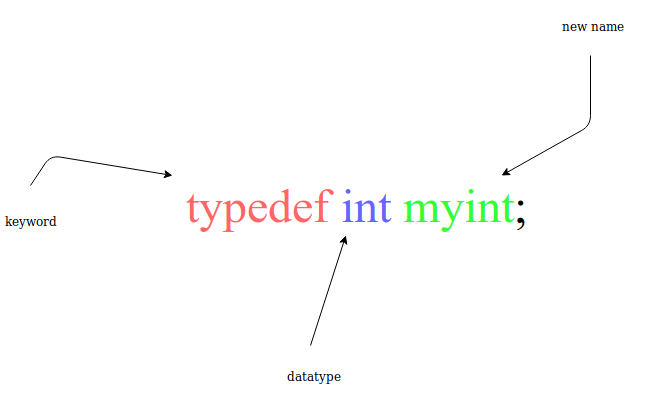×

Search anything:

# Working with Typedef in C

#### Software Engineering C typedefGet this book -> Problems on Array: For Interviews and Competitive Programming

typedef is a keyword in C programming language,which stands for type definition. Typedefs can be used both to:

• provide more clarity to your code
• make it easier to make changes to the underlying data types that you use
• Typedefs can make your code more clear and easier to modify.

One thing to keep in mind when using typedefs is that the underlying type might matter; avoid typedefs, or make them easy to find, when it's important to know the underlying data type

• How to use typedef?
• Why and when to use typedef?
• Difference between typedef and define

## Syntax

The Syntax of using type def is as follows:

``````typedef <expression you want to replace> <your new name>
``````

Example to replace int with myint:

``````typedef int myint
``````

Following this, you may use myint in place of int like:

``````myint data = 123;
``````Example:

``````#include<stdio.h>
typedef int myint;
void main( )
{
myint integer = 10;
printf("%d",integer);
}
Output
10
``````

## What are the applications of typedef?

• typedef can be used to give a name to user defined data type.
``````#include<stdio.h>
typedef struct vehicle
{
char name;
char  model;
}car;

void main( )
{
car c1;
scanf("%s", c1.name);               //BMW
scanf("%s", c1.model);              // S7
printf("\nCar name: %s\n",c1.name);
printf("\nCar Model: %s\n",c1.model);

}
Output
Car name: BWM
Car Model: S7
``````
• typedef can be used to give an name to pointers also.
``````#include<stdio.h>
typedef int* IntPtr;

void main( )
{
IntPtr x;
printf("%p",x);                    // print's 0x7ffdbefa34cc
}
``````

## Difference between typedef and #define

• A typedef is the (partial storage-class specifier) compiler directive mainly use with user-defined data types (structure, union or enum) to reduce their complexity and increase the code readability and portability.
``````typedef unsigned int UnsignedInt;
``````
• A #define is a pre-processor directive and it replaces the value before compiling the code. #define will just copy-paste the definition values at the point of use, while typedef is actual definition of a new type.
``````#define Value 10
``````

## Question

#### typedef which of the following may create problem in the program?

;
Arithmetic operators
printf/scanf
All of the mentioned.#### Bharat Arya

Computer Science Student at University of Petroleum and Energy Studies, Dehradun and Intern at OpenGenus

Working with Typedef in C